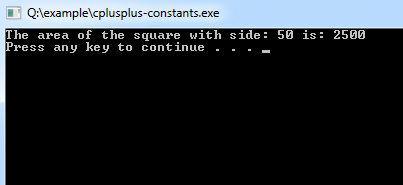## C++ Programming Tutorial Index

Constants are like a variable except that once defined, their value never changes during the program's execution. In this tutorial, you will learn about Constants in C++.

## What are Constants?

Constants refer to as fixed values; Unlike variables whose value can be changed, constants - as the name implies, do not change; They remain constant. A constant must be initialized when created, and new values cannot be assigned to it later.

• Constants are also called literals.
• Constants can be any of the data types.
• It is considered best practice to define constants using only upper-case names.

## Constant Definition in C++

There are two other different ways to define constants in C++. These are:

• By using the `const` keyword
• By using `#define` preprocessor

### Constant Definition by Using `const` Keyword

Syntax:

``const type constant_name;``

Example:

``````#include <iostream>
using namespace std;

int main()
{
const int SIDE = 50;
int area;
area = SIDE*SIDE;
cout<<"The area of the square with side: " << SIDE <<" is: " << area << endl;
system("PAUSE");
return 0;
}``````

Program Output:It is also possible to put const either before or after the type.

``int const SIDE = 50;``

or

``const int SIDE = 50;``

### Constant Definition by Using `#define` preprocessor

Syntax:

``#define constant_name;``

Example:

``````#include <iostream>
using namespace std;

#define VAL1 20
#define VAL2  6
#define Newline '\n'

int main()
{
int tot;
tot = VAL1 * VAL2;
cout << tot;
cout << Newline;
}``````